# Intermediate Geometry : How to find the endpoints of a line segment

## Example Questions

1 2 4 Next →

### Example Question #51 : Midpoint Formula

A line segment on the coordinate plane has an endpoint at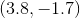; its midpoint is at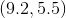.

True or false: Its other endpoint is located at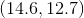.

False

True

True

Explanation:

The midpoint of a line segment with endpoints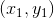and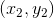is located at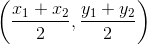.

Therefore, set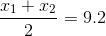and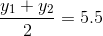In the first equation, set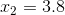and solve for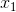: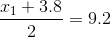Multiply both sides by 2: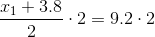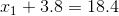Subtract 3.8 from both sides: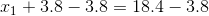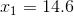In the second equation, set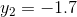and solve for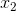: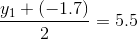Multiply both sides by 2: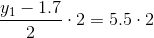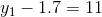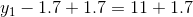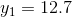The other endpoint is indeed at, so the statement is true.